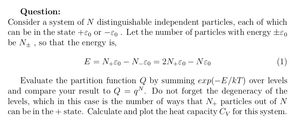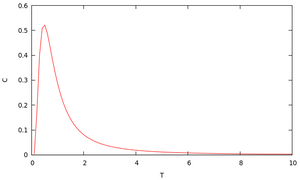Solved Problems in Physics, Physical Chemistry, and Computer Simulation Projects for College and Graduate Students.# Partition Function and Heat Capacity of Two-State Particles

Regular price
\$3.00
Sale price
\$3.00
Tax included.

Question:

Consider a system of N distinguishable independent particles, each of which can be in the state +ε0 or −ε0 . Let the number of particles with energy ±ε0 be N± , so that the energy is,

E = N+ ε0 − N- ε0 = 2N+ ε0 − N ε0

Evaluate the partition function Q by summing exp(−E/kT ) over levels and compare your result to Q = qN. Do not forget the degeneracy of the levels, which in this case is the number of ways that N+ particles out of N can be in the + state. Calculate and plot the heat capacity CV for this system.

By purchasing this product, you will get the step by step solution of the above problem in pdf format and the corresponding latex file where you can edit the solution. I am also available to help  you with any possible question you may have.